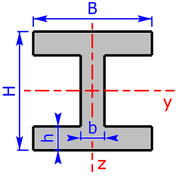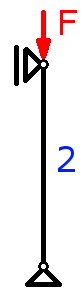Diese Seite auf Deutsch: Knicken von Stäben (Euler und Tetmajer)

# Buckling of Columns (Euler and Tetmajer) - The four Euler Buckling Modes

With this online calculator, the safety against buckling, the critical load/force and the buckling stress of columns can be calculated whereby the load and the cross-sectional shape must be known. The four Euler buckling modes are taken into account. It is calculated either according to Tetmajer (inelastic range) or according to Euler (elastic range). After the calculator you will find the formulas that this program uses.

The two construction steels S235 (St37) and S355 (St52) are available as material. It is also possible to enter individual material parameters. The minimum axial area moment of inertia and the cross sectional area can either be calculated approximately or entered directly if these values are known from tables.

## Calculator for the Buckling Load & Safety against Buckling

By default, one can calculate the safety against buckling for an I-beam (I100) from construction steel S235. The buckling force is 10 kN and the buckling length is 2 m.

 cross section circle pipe semicircle rectangle re. + bore rectangle-pipe I/H-section U/C-section L-section own section hexagon octagon height H mm width B mm height h mm width b mm
 material S235 (St37) S355 (St52) force F kN buckling mode 1 2 3 4 factor β * length l mstress σex N/mm2 buckling stress σK N/mm2
 safety S buckling force FK kN

### For experts: input of individual & output of special values

 E-modulus N/mm2 coeff. a coeff. b *** coeff. c
 Re N/mm2 λg Imin ** cm4 A ** mm2

* Is automatically entered by selecting an Euler buckling mode, can be changed any time.

** To enter these values, select under cross section -> other sections-> "own section".

*** Coefficient b must normally have a negative sign in this calculator!

### Explanation of the abbreviations

 Dm diameter in mm β column effective length factor needed for calculation of lk; this value depends on Euler buckling mode l length of column in m lk buckling length; lk = β × l; in m σex existing stress in the column; σex = F ÷ A; in N/mm² σK buckling stress in N/mm² FK buckling force = maximum or critical force/load; in kN S safety against buckling; S = FK ÷ F Re yield strength in N/mm² λg limit slenderness: determines whether in the present case it must be calculated by Tetmajer (inelastic range) or by Euler (elastic range) Imin minimum axial area moment of inertia in cm4 A cross sectional area of the column in mm² a, b, c coefficients for the Tetmajer-equation; coefficient b must normally have a negative sign in this calculator!

### Manual

• The following cross sectional areas are avaible:
• circle
• pipe/hollow circular
• semi-circle
• rectangle-section
• rectangle with bore
• rectangle-pipe
• I/H-section (I/H-beam)
• U/C-section (U/C-beam)
• L-section (isosceles)
• hexagon/six-sided figure
• octagon/eight-sided figure
• own section
• In mechanical engineering, the safety has to be about twice as high as in steel construction. The calculator uses the minimum safety of steel construction. You always have to plan for relatively large safeties since the calculations apply to an ideal column only:
• The force must act exactly in the column axis and that at right angles to the cross sectional area.
• The column is assumed to be homo­geneous.
• There must be no other forces and moments, such as wind loads.
• The cross sectional areas must always be symmetrical to the two coordinate axes.
• Accuracy can not be guaranteed - for corrections or additions please use my contact form!

Note:

In the elastic range (Euler), all steels buckles at the same load! In this case there is no difference if you use a S355 or a S235!

## How do you do the calculation against buckling?

General example: The individual calculation steps including formulas are given.

### The four Euler Buckling Modes - factor β needed for calculation of lk

First, you have to decide which of the four Euler buckling modes is present. The following picture shows the four Euler buckling modes depending on the boundary conditions:

• Case 1: firm fixing at the bottom, upper end free to move laterally; β = 2
• Case 2: below fixed bearing, above floating bearing = both ends hinged (free to rotate); β = 1; this is the most common case in practice
• Case 3: firm fixing at the bottom, above floating bearing (= hinged); β = 0.699
• Case 4: firm fixing at the bottom, upper end guided in longitudinal slot; β = 0.5

The following relationship applies: lk = β × l

lk is called the buckling length, l is the length of the column. Depending on the boundary conditions, this results in different buckling lengths.

### Formulas for slenderness, buckling stress and buckling force

All letters used in these formulas are explained immediately after the calculator!

First, you have to calculate the ratio of slenderness λ:

Now, the limit slenderness λg of the material used is needed. For a construction steel S235 (previously St37) this value is 105, for the material S355 (St52) it is 85.

If the computed ratio of slenderness λ is greater than λg, it is calculated according to Euler (elastic range), otherwise according to Tetmajer (inelastic range).

The following table shows the formulas for the buckling stress σK and for the buckling force FK:

#### Tetmajer (inelastic section)

E is the so-called modulus of elasticity. For steel this value is approx. 210000 N/mm².

Values for the coefficients of the Tetmajer-equation:

for S235 (St37): a = 310 and b = -1.14

for S355 (St52): a = 335 and b = -0.62

In both cases coefficient c is 0.

### Formula for Safety against buckling

Finally, the safety S against buckling is calculated: